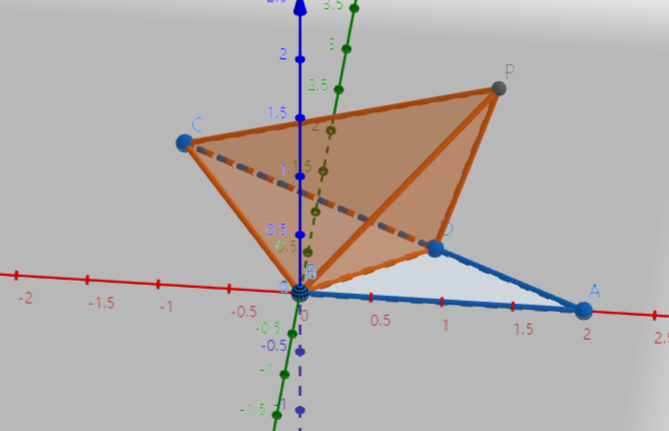# SAT1000 - P606

Geometry Level pendingAs shown above, in $\triangle ABC$, $AB=BC=2$, $\angle ABC = \dfrac{2\pi}{3}$.

If $P$ is outside plane $ABC$ and point $D$ is on segment $AC$, so that $PD=DA, PB=BA$, then find the maximum volume of pyramid $PBCD$.

Let $V$ denote the volume of $PBCD$, submit $\lfloor 10000V \rfloor$.

Have a look at my problem set: SAT 1000 problems

×